# Understanding computer graphics algorithms. Part 1 – Starfield Simulation

With this short note, I want to start a series of articles on computer graphics algorithms. Moreover, not the hardware subtleties of the implementation of this very graphics, but the algorithmic component.

I will act according to the following principle: I take some graphic effect (from a demo, program, game – it doesn’t matter) and try to implement the same effect in the simplest and most understandable way, explaining what, how and why it was done that way.

The Python language and the PyGame library will be used as the basis for graphics output. With this set, you can very easily display something on the screen, make animation, etc. without being distracted by the technical details of the implementation.

For the basic template of the program, I will take the following code:

import pygame

SX = 800
SY = 800

pygame.init()
screen = pygame.display.set_mode((SX, SY))
running = True

while running:
for event in pygame.event.get():
if event.type == pygame.QUIT:
running = False

# Здесь будет алгоритмическая часть

pygame.display.flip()

pygame.quit()


This is a small blank that allows you to create a window for displaying graphics, and also forms an endless loop that reproduces the animation frame displayed on the screen – the so-called game loop.

I will not dwell on the above code, I think everything is as clear as possible here. Let’s get straight to the point.

What can be taken from graphic effects to start with, so that it is both as simple and as beautiful as possible?

A long time ago there was a class of programs called “screen savers”. These are small programs that showed simple animations and ran on a timer when the user did not press any keys or touch the mouse. They still exist, but now they carry more aesthetic functionality than practical ones. In the era of cathode ray tube monitors, such programs helped prevent the phosphor inside the kinescope from burning out.

If a static image with a minimum of changes is shown on the monitor screen for a long time, then you can get the effect that the phosphor applied to the inside of the kinescope evaporated due to overheating at certain points and left traces that did not disappear even when the monitor was turned off. Those. the image remained as if burned out on the monitor. By the way, LCD monitors and plasma panels, etc. are also subject to this effect.

To avoid such an effect, when the user was idle for a long time, a screensaver program was launched that updated the screen and displayed something changing, not giving separate parts of the screen a chance to burn out.

It turns out that the useful functionality also combined an aesthetic component, since everyone wanted to see something pleasing to the eye on their monitor.

One of these programs was a demonstration of the starry sky, where individual stars simply blinked. But even more beautiful was the flight through the stars.

Here are some examples of such screen savers and software products where they have been integrated:

Let’s take the screensaver from Windows 3.11 as a basis and try to replicate it, perhaps with a few improvements.

If we look at the animation, then we see a certain number of stars that are moving towards us. The stars are approaching gradually increasing their brightness. The scattering of stars occurs evenly relative to the center of the screen.

Let’s start with the fact that we need to somehow fix the total number of stars that we will display on the screen at the same time.

Let it be a constant NumStar and to begin with, we will process one hundred stars.

The stars need to be stored somewhere, since we are using Python, let it be a regular mutable list. Each star has some characteristics, and we will write them down in this list.

What we need to know about the star:

1. Its coordinates in space. Since we are emulating stars in 3D space, these will be X, Y, Z coordinates.

Z will be the depth of the screen, the larger it is, the further the star is.

2. A characteristic of the color of a star, it is also brightness. The farther the star is, the dimmer it will be, so the color value will be inversely proportional to the distance to the star.

You will get something like this: Stars[ [x1, y1, z1, color1], [x2, y2, z2, color2]etc.]

When a star flies towards us, it gradually moves along all three coordinates X, Y, Z, and someday it will fly out of the screen. A star can fall out of our field of view if it exceeded the coordinates on the plane of our window in X or Y, and also if it flew too close to us and switched to negative coordinates along the Z axis. At this moment, you need to make a new star instead of this star , so as not to waste values ​​that we will never see.

The best way to do this is to reset all the properties of the star to some initial values.

Since we need the stars to fly towards us evenly relative to the center of the screen, we will decide for ourselves that the center of our window will be the center of the coordinate system, which of course does not coincide with the coordinates provided by PyGame, but this is easily substituted.

For a uniform random spread of the coordinates of stars along the plane of our window, we apply the following approach: we know the width and height of the window (these are SX and SY), so we will receive new coordinates as:

X = random.randint(0, SX) - SX // 2

Y = random.randint(0, SY) - SY // 2

Those. we get a random number between 0 and the width or height of our window, and then divide it by 2 to get a random number between “-half the window” and “+half the window”. X and Y coordinates are ready.

The Z-coordinate is given more simply, each new star appears at the maximum distance from us. For ease of calculation, let the maximum screen depth be 256.

That’s why Z = 256.

And the color of the new star remains, but since it is far away, let the star be not visible at first, i.e. let’s give it color 0.

color = 0

As the star approaches us along the Z axis, its brightness will increase. And increase from 0 to 255.

An approximate scheme of the algorithm is as follows:

Before the main animation cycle, we perform the initial initialization of all the stars.

The animation will consist of the following steps:

• clear the screen

• we bring out the stars;

• calculate new coordinates;

• repeat.

To display the star already in screen coordinates, we need to convert 3D coordinates to 2D, and preferably in perspective projection.

Let’s try to theoretically analyze how to do this.

Firstly, what is a perspective projection – this is when, to build a projection, we need a certain point – the center of the projection, a ray comes out of it, which intersects the object for which the projection is being built and a certain plane on which the object is projected. This method allows you to display an object on a plane, taking into account its perspective distortions, i.e. the distant parts will be smaller than the near ones.

In the figure below, I will present my star in X, Y, Z coordinates. Now only two axes Y and Z are considered, for the X and Z axes everything will be exactly the same. The projection center will be located at the origin. A virtual ray emerges from the projection center, which intersects the star and further intersects the plane of my screen, on which the star will be displayed in a 2D view (there will no longer be a Z axis here).

This can be compared to a flashlight that shines from a central point and a star casts a shadow on a certain wall (my screen).

The point on the screen will have coordinates. Since we are now considering the Y and Z axes, X is not taken into account.

I need to calculate the coordinates.

Here it is appropriate to recall the school geometry course and the triangle similarity theorem, which states that the ratios of similar sides of similar triangles are equal. Accordingly, the following statement will be true: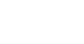Based on this, we calculate: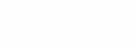Let’s place the plane for display at the farthest point of our virtual field along the Z axis, at coordinate 256. As a result, we get formulas for calculating the screen coordinates of our stars on the plane in the following form: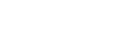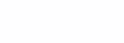But since we have slightly modified our center of coordinates, compared to what PyGame suggests (and it considers the origin from the upper left corner of the window), we need to convert the received coordinates to the PyGame coordinate system.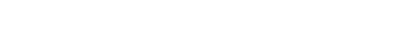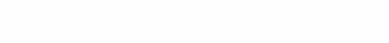As a result, when the star moves to the center of coordinates along the Z axis, it will move up along the Z axis, and the effect of stars scattering from the center of the screen will occur.

Let’s make the movement of all stars with the same speed – speed. Let’s choose the speed experimentally, I got it equal to 0.09, for the slow and beautiful movement of the stars.

In the loop, for each star, we decrease its Z coordinate and recalculate X and Y. If the Z coordinate has become less than or equal to 0 or the star has flown out of any of the side boundaries of the screen along X or Y, then we generate a new star instead of the old one.

Simultaneously with the decrease in the Z coordinate, we increase the color value of the star so that when it approaches us, the brightness increases. Empirically, the increase in brightness, for the most pleasant picture, I got in increments of 0.15.

And the final code will look like this:

import pygame
import random

SX = 800
SY = 800

pygame.init()
screen = pygame.display.set_mode((SX, SY))
running = True

NumStar = 100			# Общее количество звезд
speed = 0.09			# Скорость полета звезд
stars = []			  # Список, содержащий звезды
# каждая звезда состоит из X-координаты, Y-координаты,
# расстояния по Z (дальность до звезды), цвет

# -----------------------------------------------------------------------------------
# Функция генерации новой звезды.
# -----------------------------------------------------------------------------------
def new_star():
star = [random.randint(0, SX) - SX // 2, random.randint(0, SY) - SY // 2, 256, 0]
return star

# -----------------------------------------------------------------------------------
for i in range(0, NumStar):		# Заполняем список новыми сгенерированными звездами.
stars.append(new_star())

while running:
for event in pygame.event.get():
if event.type == pygame.QUIT:
running = False

screen.fill((0, 0, 0))		    # Очищаем экран
x = y = 0
for i in range(0, NumStar):		# Цикл по всем звездам.
s = stars[i]			    		# Запоминаем характеристики звезды из списка
# Вычисляем текущие координаты звезды
x = s * 256 / s
y = s * 256 / s
s -= speed        			# Изменяем ее координату по Z

# Если координаты вышли за пределы экрана - генерируем новую звезду.
if s <= 0 or x <= -SX // 2 or x >= SX // 2 or y <= -SY // 2 or y >= SY // 2:
s = new_star()

if s < 256:			# Если цвет не достиг максимума яркости, увеличиваем цвет.
s += 0.15

if s >= 256:			# Если вдруг цвет стал больше допустимого, то выставляем его как 255
s = 255

stars[i] = s				# Помещаем звезду обратно в список звезд.

# Отображаем звезду на экране.
x = round(s * 256 / s) + SX // 2
y = round(s * 256 / s) + SY // 2
pygame.draw.circle(screen, (s, s, s), (x, y), 3)

pygame.display.flip()

pygame.quit()


We get an analogue of the “ancient” screen saver in the Python language. This algorithm and graphical effect is one of the simplest, but contains some interesting mathematics and geometric transformations.